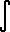Home    |    Teacher    |    Parents    |    Glossary    |    About UsEmail this page to a friendResources· Cool Tools · Formulas & Tables · References · Test Preparation · Study Tips · Wonders of MathSearchProof: Integral cosh(x) (Math | Calculus | Integrals | Table Of | cosh x)

 Discussion ofcosh x dx = sinh x + C.

## 1. Proof

Strategy: Use definition of cosh.
 cosh x = ex + e-x  2cosh x dx =ex + e-x  2 dx
= (1/2)ex dx + (1/2)e-x dx
solve left equation
= (1/2) ex + (1/2)e-x dx

set
u = - x
then we find
du = - dx

substitute du= - dx, u= - x

= (1/2) ex - (1/2)- e-x dx
= (1/2) ex - (1/2)eu du

solve the right integral
= (1/2) ex - (1/2) eu + C

substitute back u= - x
= (1/2) ex - (1/2) e-x + C  = ex - e-x  2 + C
which by definition
= sinh x + C
Q.E.D.